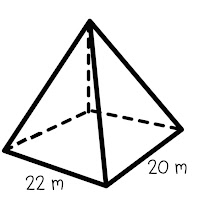Volume Quiz
You will be reviewing all of your work on volume of spheres, cones, cubes, rectangular prisms, and cylinders.
What is the volume of a cone with a diameter of 12 inches and a height of 16 inches. Round to the nearest tenth and write the number answer only. *
1 point
The volume of a sphere 800 cubic feet. Find the length of the radius. Round to the nearest tenth and write the number answer only. *
1 point
What is the volume of the sphere? Round to the nearest tenth and write the number answer only. *
1 point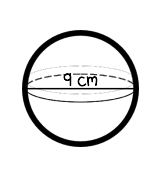The base of the rectangular prism has an area of 25 cubic feet. The height is 12 feet. What is the volume? Round to the nearest tenth and write the number answer only. *
1 point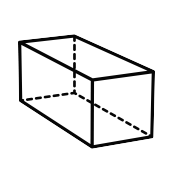find the volume of the cone. *10 yards is the slant height.* Round to the nearest tenth and write the number answer only. *
1 point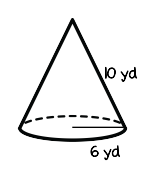The volume of a pyramid is 300 cubic centimeters. If the height is 14 cm. and the length is 5 cm., what is the width? Round to the nearest tenth and write the number answer only. *
1 point
the solid has a radius of 7 inches and a total height of 20 inches. Find the volume. Round to the nearest tenth and write the number answer only. *
1 point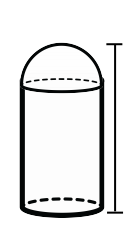A cardboard box is used to ship golf balls to different stores. The box is 12 in/ by 48 in. by 60 in. If each golf ball has a radius of 0.84 inches, how many golf balls will fit inside the box? Round to the nearest whole number and write the number answer only. *
1 point
The height of the pyramid is 36 meters. Find the volume. Round to the nearest tenth and write the number answer only. *
1 point